University Physics Volume 2

# Challenge Problems

University Physics Volume 2Challenge Problems

### Challenge Problems

75.

A copper wire of length L is fashioned into a circular coil with N turns. When the magnetic field through the coil changes with time, for what value of N is the induced emf a maximum?

76.

A 0.50-kg copper sheet drops through a uniform horizontal magnetic field of 1.5 T, and it reaches a terminal velocity of 2.0 m/s. (a) What is the net magnetic force on the sheet after it reaches terminal velocity? (b) Describe the mechanism responsible for this force. (c) How much power is dissipated as Joule heating while the sheet moves at terminal velocity?

77.

A circular copper disk of radius 7.5 cm rotates at 2400 rpm around the axis through its center and perpendicular to its face. The disk is in a uniform magnetic field $B→B→$ of strength 1.2 T that is directed along the axis. What is the potential difference between the rim and the axis of the disk?

78.

A short rod of length a moves with its velocity $v→v→$ parallel to an infinite wire carrying a current I (see below). If the end of the rod nearer the wire is a distance b from the wire, what is the emf induced in the rod?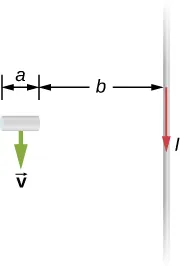79.

A rectangular circuit containing a resistance R is pulled at a constant velocity $v→v→$ away from a long, straight wire carrying a current $I0I0$ (see below). Derive an equation that gives the current induced in the circuit as a function of the distance x between the near side of the circuit and the wire.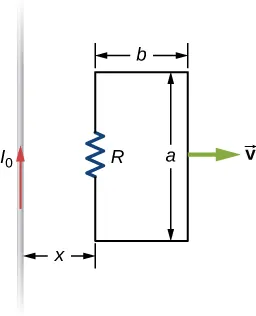80.

Two infinite solenoids cross the plane of the circuit as shown below. The radii of the solenoids are 0.10 and 0.20 m, respectively, and the current in each solenoid is changing such that $dB/dt=50.0T/s.dB/dt=50.0T/s.$ What are the currents in the resistors of the circuit?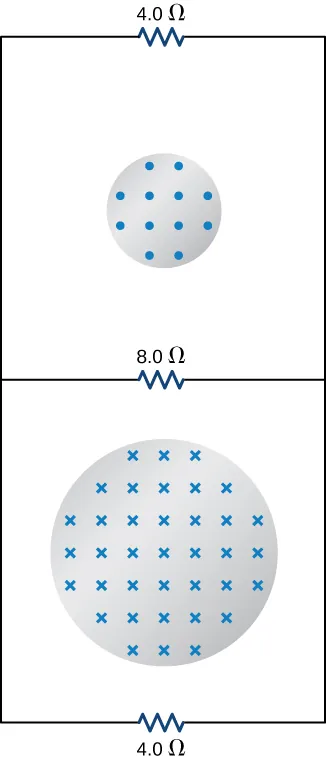81.

An eight-turn coil is tightly wrapped around the outside of the long solenoid as shown below. The radius of the solenoid is 2.0 cm and it has 10 turns per centimeter. The current through the solenoid increases according to $I=I0(1−e−αt),I=I0(1−e−αt),$ where $I0=4.0AI0=4.0A$ and $α=2.0×10−2s−1.α=2.0×10−2s−1.$ What is the emf induced in the coil when (a) $t=0t=0$, (b) $t=1.0×102s,t=1.0×102s,$ and (c) $t→∞?t→∞?$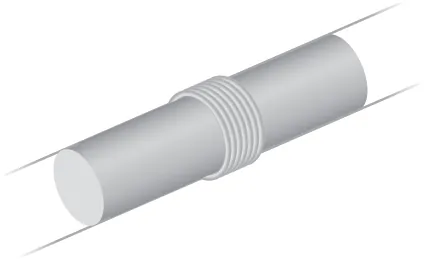82.

Shown below is a long rectangular loop of width w, length l, mass m, and resistance R. The loop starts from rest at the edge of a uniform magnetic field $B→B→$ and is pushed into the field by a constant force $F→.F→.$ Calculate the speed of the loop as a function of time.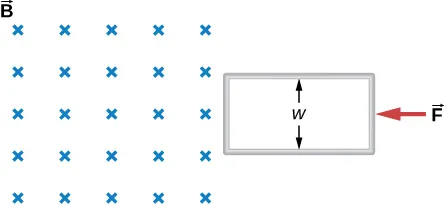83.

A square bar of mass m and resistance R is sliding without friction down very long, parallel conducting rails of negligible resistance (see below). The two rails are a distance l apart and are connected to each other at the bottom of the incline by a zero-resistance wire. The rails are inclined at an angle $θθ$, and there is a uniform vertical magnetic field $B→B→$ throughout the region. (a) Show that the bar acquires a terminal velocity given by $v=mgRsinθB2l2cos2θ.v=mgRsinθB2l2cos2θ.$ (b) Calculate the work per unit time done by the force of gravity. (c) Compare this with the power dissipated in the Joule heating of the bar. (d) What would happen if $B→B→$ were reversed?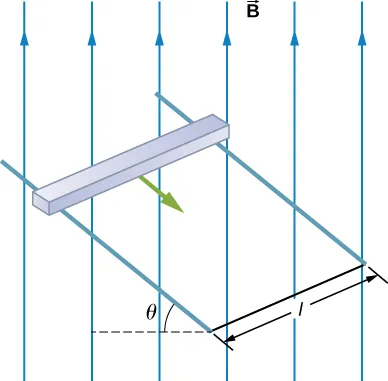84.

The accompanying figure shows a metal disk of inner radius $r1r1$ and other radius $r2r2$ rotating at an angular velocity $ω→ω→$ while in a uniform magnetic field directed parallel to the rotational axis. The brush leads of a voltmeter are connected to the dark’s inner and outer surfaces as shown. What is the reading of the voltmeter?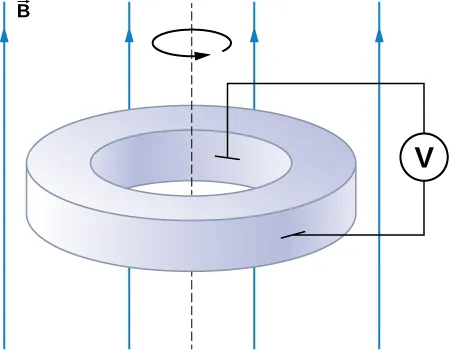85.

A long solenoid with 10 turns per centimeter is placed inside a copper ring such that both objects have the same central axis. The radius of the ring is 10.0 cm, and the radius of the solenoid is 5.0 cm. (a) What is the emf induced in the ring when the current I through the solenoid is 5.0 A and changing at a rate of 100 A/s? (b) What is the emf induced in the ring when $I=2.0AI=2.0A$ and $dI/dt=100A/s?dI/dt=100A/s?$ (c) What is the electric field inside the ring for these two cases? (d) Suppose the ring is moved so that its central axis and the central axis of the solenoid are still parallel but no longer coincide. (You should assume that the solenoid is still inside the ring.) Now what is the emf induced in the ring? (e) Can you calculate the electric field in the ring as you did in part (c)?

86.

The current in the long, straight wire shown in the accompanying figure is given by $I=I0sinωt,I=I0sinωt,$ where $I0=15AI0=15A$ and $ω=120πrad/s.ω=120πrad/s.$ What is the current induced in the rectangular loop at (a) $t=0t=0$ and (b) $t=2.1×10−3s?t=2.1×10−3s?$ The resistance of the loop is $2.0Ω.2.0Ω.$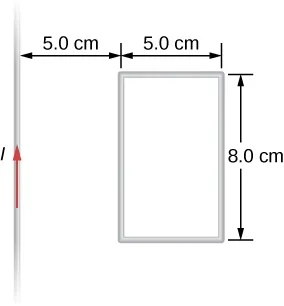87.

A 500-turn coil with a $0.250-m20.250-m2$ area is spun in Earth’s $5.00×10−5T5.00×10−5T$ magnetic field, producing a 12.0-kV maximum emf. (a) At what angular velocity must the coil be spun? (b) What is unreasonable about this result? (c) Which assumption or premise is responsible?

88.

A circular loop of wire of radius 10 cm is mounted on a vertical shaft and rotated at a frequency of 5 cycles per second in a region of uniform magnetic field of $2×10−4T2×10−4T$ perpendicular to the axis of rotation. (a) Find an expression for the time-dependent flux through the ring (b) Determine the time-dependent current through the ring if it has a resistance of $10Ω10Ω$.

89.

A long solenoid of radius $aa$ with $nn$ turns per unit length is carrying a time-dependent current $I(t)=I0sinωtI(t)=I0sinωt$ where $I0I0$ and $ωω$ are constants. The solenoid is surrounded by a wire of resistance R that has two circular loops of radius b with $b>ab>a$. Find the magnitude and direction of current induced in the outer loops at time $t=0t=0$.

90.

A rectangular copper loop of mass 100 g and resistance $2.0Ω2.0Ω$ is in a region of uniform magnetic field of strength $3.0T3.0T$ that is perpendicular to the area enclosed by the ring and horizontal to Earth’s surface (see below). The width of the loop is $a=25cma=25cm$. The loop is let go from rest when it is at the edge of the nonzero magnetic field region. (a) If the loop were long enough for it to reach terminal velocity before exiting the region of magnetic field, what would be its terminal velocity? (b) Find an expression for the speed as a function of time while the top of the loop is still in the magnetic field.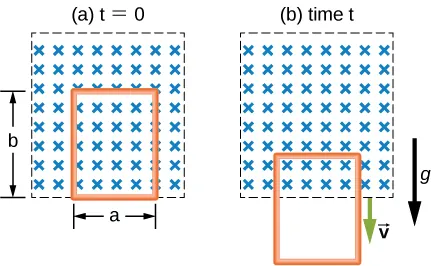91.

A metal bar of mass m slides without friction over two rails a distance D apart in the region that has a uniform magnetic field of magnitude $B0B0$ and direction perpendicular to the rails (see below). The two rails are connected at one end to a resistor whose resistance is much larger than the resistance of the rails and the bar. The bar is given an initial speed of $v0v0$. It is found to slow down. How far does the bar go before coming to rest? Assume that the magnetic field of the induced current is negligible compared to $B0B0$.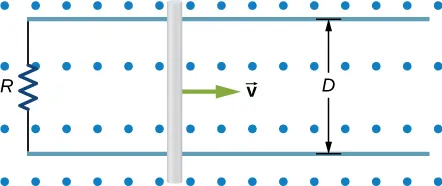92.

A time-dependent uniform magnetic field of magnitude B(t) is confined in a cylindrical region of radius R. A conducting rod of length 2D is placed in the region, as shown below. Show that the emf between the ends of the rod is given by $dBdtDR2−D2dBdtDR2−D2$. (Hint: To find the emf between the ends, we need to integrate the electric field from one end to the other. To find the electric field, use Faraday’s law as “Ampère’s law for E.”)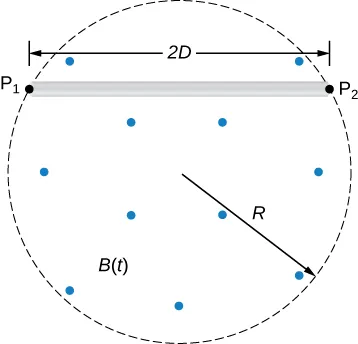Order a print copy

As an Amazon Associate we earn from qualifying purchases.